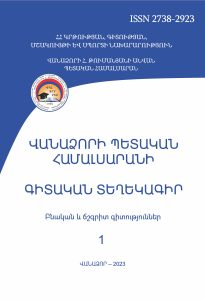# On a Method of Solving the Thermal Conductivity Equation

Scientific Proceedings of  Vanadzor State University Natural and Exact Sciences (ISSN 2738-2923)

2023 vol 1

On a Method of Solving the Thermal Conductivity Equation of an Inhomogeneous Isotropic Body

Sergey Azaryan

SummaryKey words: coefficient of internal heat conductivity, classical problems, Cauchy problem, small parameter method, second-order partial differential equation, inhomogeneity function

Classical problems in various formulations have been solved by many authors (Cauchy, Dirichle, Neumann and others) for isotropic homogeneous bodies in the theory of elasticity. Such boundary value problems have been reduced to solving differential equations with constant coefficients [1-3; 5].

The paper considers the problem of thermal conduction of an inhomogeneous isotropic body, which leads to the solution of a second-order partial differential equation with variable coefficients (1.3). With sufficient smoothness of the variable coefficients, through transformations, as well as the characteristic equation, this second-order differential equation in a given region can be reduced to one of the following forms; elliptic, hyperbolic and parabolic. Here, the boundary value problem is reduced to solving a second order differential equation with variable parabolic coefficients.

A small physical parameter is introduced, and a recurrent sequence of boundary value problems for the Cauchy boundary value problem is obtained in the article. When solving these boundary value problems, the general solution is represented as a series by formula (2.2) and under certain conditions depending on δ and σ the convergence of the series is proved and the solution is reduced to (5.4). In case of varying the inhomogeneity function f (x,y,z), a small physical parameter δ and a constant σ, a set of inhomogeneous isotropic bodies is obtained, the results of which will be compared with the results of homogeneous isotrpic bodies.

PDF